# Spatial data in SQL Server 2008

## Combining Geometry instances

We'll now go a step further and show methods that allow two or more instances to interact with one another in order to produce a new Geometry.

For my baseline, I'll use two Polygons that overlap each other:

``````DECLARE @g geometry
= 'POLYGON((10 10, 40 10, 40 40, 10 40, 10 10))'
DECLARE @h geometry
= 'POLYGON((30 30, 50 30, 50 50, 30 50, 30 30))'
``````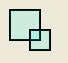## STDifference

STDifference() returns a new instance consisting of all points from the base instance that do not contain points from the parameter instance.

`````` SELECT @g.STDifference(@h).ToString();
``````

Result:

``````POLYGON ((10 10, 40 10, 40 30, 30 30, 30 40, 10 40, 10 10))
``````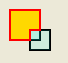## STIntersection

STIntersection() returns a new instance containing only the points that are in common between the base instance and the parameter instance.

``````SELECT @g.STIntersection(@h).ToString();
``````

Result:

``````POLYGON ((30 30, 40 30, 40 40, 30 40, 30 30))
``````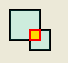## STSymDifference

STSymDifference() returns a new instance containing only the points that are unique to both the base instance and the parameter instance (i.e., it excludes the points that STIntersection() would return).

In this case, the set of points is actually two different Polygons. Because STSymDifference() needs to return a single instance of something, it will wrap those two Polygons into a collection (MultiPolygon).

``````SELECT @g.STSymDifference(@h).ToString();
``````

Result:

``````MULTIPOLYGON (((40 30, 50 30, 50 50, 30 50, 30 40, 40 40, 40 30)),
((10 10, 40 10, 40 30, 30 30, 30 40, 10 40, 10 10)))
``````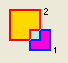## STUnion

STUnion() returns a new instance containing all of the points of the base instance and the parameter instance merged together.

``````SELECT @g.STUnion(@h).ToString();
``````

Results:

``````POLYGON ((10 10, 40 10, 40 30, 50 30, 50 50, 30 50,
30 40, 10 40, 10 10))
``````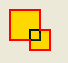## Blended Types

The instance methods described above do not work just for Polygons. You can actually use them on different types, or even collections of different types.

For instance, if we look at the results of using a LineString as the base instance and a Polygon as the parameter instance, STDifference() will return a MultiLineString constisting of the points from the original LineString that do not lie within the Polygon:

``````DECLARE @g geometry = 'LINESTRING(9 9, 40 40)'
DECLARE @h geometry = 'POLYGON((15 15, 15 30, 30 30, 30 15, 15 15))'
SELECT @g.STDifference(@h).ToString();
``````

Results:

``````MULTILINESTRING ((40 40, 30 30), (15 15, 9 9))
``````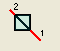STIntersection() will return the points from the original LineString that do lie within the Polygon:

``````SELECT @g.STIntersection(@h).ToString();
``````

Results:

``````LINESTRING (30 30, 15 15)
``````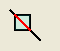STUnion() cannot determine a single common Geometry type, so it will return a mixed collection of types:

``````SELECT @g.STUnion(@h).ToString();
``````

Results:

``````GEOMETRYCOLLECTION
(
LINESTRING (40 40, 30 30),
POLYGON ((15 15, 30 15, 30 30, 15 30, 15 15)),
LINESTRING (15 15, 9 9)
)
``````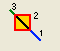## You might also like...

### Jason FollasJason Follas is the President of the Northwest Ohio .NET User Group (NWNUG) and is a Technical Architect for Perficient...

Interested in writing for us? Find out more.

### Contribute

Why not write for us? Or you could submit an event or a user group in your area. Alternatively just tell us what you think!

### Our tools

We've got automatic conversion tools to convert C# to VB.NET, VB.NET to C#. Also you can compress javascript and compress css and generate sql connection strings.

“If debugging is the process of removing software bugs, then programming must be the process of putting them in.”